# Short Answer Type Questions- Coordinate Geometry Notes | Study Mathematics (Maths) Class 9 - Class 9

## Class 9: Short Answer Type Questions- Coordinate Geometry Notes | Study Mathematics (Maths) Class 9 - Class 9

The document Short Answer Type Questions- Coordinate Geometry Notes | Study Mathematics (Maths) Class 9 - Class 9 is a part of the Class 9 Course Mathematics (Maths) Class 9.
All you need of Class 9 at this link: Class 9

Question 1. What are the coordinates of A, B, C and D in the following figure?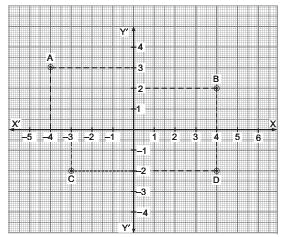Solution: The coordinates of A are (–4, 3).
The coordinates of B are (4, 2).
The coordinates of C are (–3, –2).
The coordinates of D are (4, –2).

Question 2. Look at the following figure and answer the following:
(i) What is the abscissa of P?
(ii) What is the ordinate of Q?
(iii) What is the ordinate of R?
(iv) What is the abscissa of S?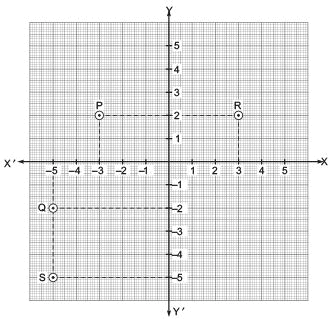Solution: (i) The abscissa of P is –3.
(ii) The ordinate of Q is –2.
(iii) The ordinate of R is 2.
(iv) The abscissa of S is –5.

Question 3. Look at the following figure and answer the following: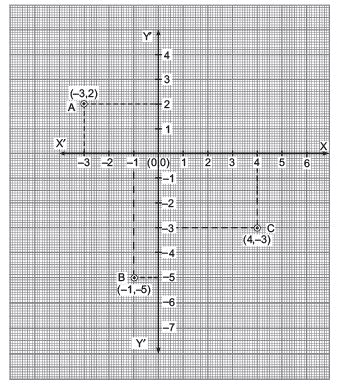(i) Which point is having its ordinate as (–5)?
(ii) Which point is having abscissa as 4?
(iii) What are the coordinates of origin?
(iv) Which point is having abscissa as (–3)?
Solution: (i) The point B is having its ordinate as (–5).
(ii) The point C is having its abscissa as 4.
(iii) The coordinates of O are (0, 0).
(iv) The point A is having abscissa as (–3).

Question 4. Look at the following figure and answer the following:
(i) Which two points have the same abscissa?

(ii) Which two points have the same ordinate?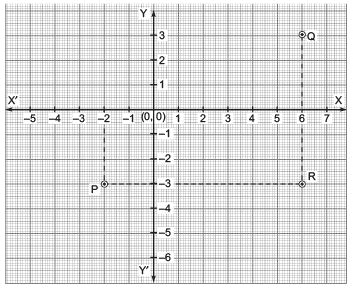Solution: (i) ∵ The abscissa of the point Q is 6.
The abscissa of the point R is 6.
∴The points Q and R have the same abscissa.
(ii) ∵ The ordinate of P is (–3).
The ordinate of R is (–3).
∴ The points P and R have the same ordinate.

Question 5. Read the coordinates of the vertices of the triangle ABC in the following figure: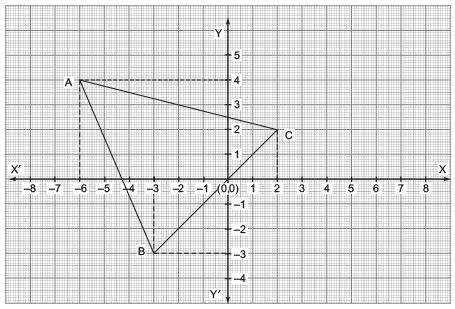Solution: The vertices of the triangle are A, B and C.
The coordinates of A are (–6, 4).
The coordinates of B are (–3, –3).
The coordinates of C are (2, 2).

Question 6. Write the coordinates of quadrilateral PQRS as shown in the following figure: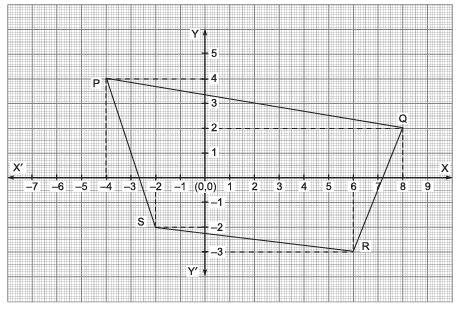Solution: The vertices of the quadrilateral are P, Q, R and S.
The coordinates of P are (–4, 4).
The coordinates of Q are (8, 2).
The coordinates of R are (6, –3).
The coordinates of S are (–2, –2).

Question 7. Write the vertices of the following quadrilateral OBCD.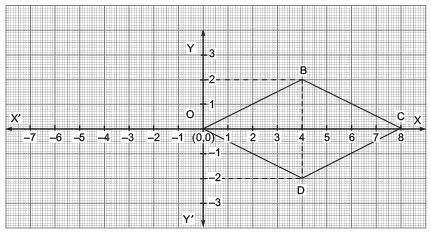Solution: The vertices of the quadrilateral are O, B, C and D.
The coordinates of O are (0, 0).
The coordinates of B are (4, 2).
The coordinates of C are (8, 0).
The coordinates of D are (4, –2).

Question 8. Draw a figure whose vertices are on the points whose coordinates are (4, 0), (–4, 0), (–4, 4), (4, 4) and (0, 2).
Solution:
Let we draw the coordinate axes XOX' and YOY'. Now plot the points (4, 0), (–4, 0), (–4, 4), (4, 4) and (0, 2) and joining them, we get a figure as shown in the figure. It is a pentagon with its vertices as A(–4, 0), B(–4, 4), C(0, 2), D(4, 4) and E(4, 0).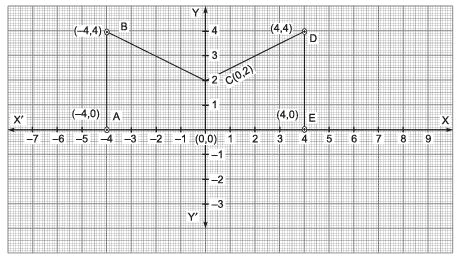Question 9. Draw a quadrilateral whose vertices are (3, 2), (2, 3), (–4, 5) and (5, –4).
Solution:
First, we draw the coordinate axes XOX' and YOY'. Now let the vertices of the required quadrilateral are A(3, 2), B(2, 3), C(–4, 5) and D(5, –4).
Therefore, plot the point A, B, C and D and join them.
We get the quadrilateral ABCD as shown in the following figure.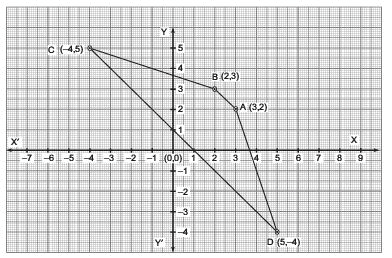Question 10. Draw a quadrilateral whose vertices are the points having coordinates as: (–3, 3), (3, 3), (3, –3) and (–3, –3). What is the special name of the quadrilateral so obtained?
Solution:
First, we draw the coordinate axes XOX' and YOY'.
Let the points corresponding to the coordinates (–3, 3), (3, 3), (3, –3) and (–3, –3) be A, B, C and D respectively. ∴ Plot the coordinates of A, B, C and D.
Now, join A, B, C and D.
We obtain a quadrilateral ABCD. The special name of the quadrilateral so obtained is the square ABCD.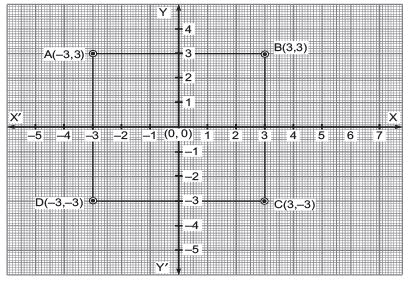Question 11. Three vertices of a rectangle are (3, 2), (–4, 2) and (–4, 5). Plot these points and find the coordinates of the fourth vertex.
Solution:
Plotting the given points we get the following figure. Name these points as A (3, 2), B (–4, 2) and C (–4, 5).
Let the rectangle be ABCD.
We have to find its fourth vertex D.
Since, the opposite sides of a rectangle are equal.
∴ The abscissa D should be equal to the abscissa of the vertex A.
⇒ The abscissa of D is 5 Thus, the coordinates of the fourth vertex D are (3, 5) as shown in the figure.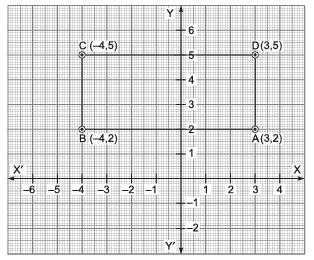The document Short Answer Type Questions- Coordinate Geometry Notes | Study Mathematics (Maths) Class 9 - Class 9 is a part of the Class 9 Course Mathematics (Maths) Class 9.
All you need of Class 9 at this link: Class 9Use Code STAYHOME200 and get INR 200 additional OFF

## Mathematics (Maths) Class 9

73 videos|352 docs|109 tests

Track your progress, build streaks, highlight & save important lessons and more!

,

,

,

,

,

,

,

,

,

,

,

,

,

,

,

,

,

,

,

,

,

;